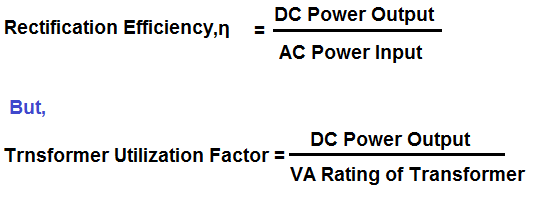# Difference between Rectification Efficiency and Transformer Utilization Factor

The main difference between Rectification Efficiency and Transformer Utilization Factor (TUF) is that former is measurement of efficiency of rectifier while latter is the measurement of extent to which transformer capacity is utilized.

Rectification Efficiency is quantitative measurement of rectifier efficiency. It is ratio of DC power output to the AC power input of the rectifier. It is usually denoted by η and expressed in percentage.Thus better the rectification efficiency (RE) more will be the DC power output for the same AC input. Lets there are two different rectifiers, say, X and Y. X is having rectification efficiency of 40.5% while Y have 81%. For an AC power input of 100 Watt, the DC power output for rectifiers X and Y will be 40.5 & 81 Watt respectively. Thus we see that, the more the rectification efficiency, the more will be conversion of AC input into DC.

In contrary, Transformer Utilization Factor (TUF) is the quantitative measurement of utilization of Transformer VA (Volt Ampere) capacity. It is ratio of DC power output to the VA rating of Transformer. It gives an idea of size of Transformer required. Note that, the size of transformer increases with its VA rating. Therefore, if the VA rating requirement increases, the size will also increase. The higher the value of TUF, the lesser will be the size of Transformer required for the rectifier and vice versa.

Let us now co-relate TUF and RE for better understanding the difference between these two parameters. For this purpose we take example of Center Tapped Full Wave Rectifier. Let the DC load of rectifier is 100 Watt.

The value of TUF and rectification ratio for Center Tapped Full Wave Rectifier is 0.672 & 81%. Since the rectification ratio is 81%, the AC input required by the rectifier to feed 100 watt load will be 123.5 watt (100/0.81 = 123.5). This means we need to provide AC input of 123 watt to the rectifier. To do this, we need transformer. So what will be the VA Rating of Transformer? You can say, it will be something around 123 VA (assuming power factor of unity). But it is not so. We don’t know the TUF. To answer, first we need to know the TUF. The TUF for Center Tapped Rectifier is 0.672. Therefore, the VA rating of required transformer will be (DC Power Output /TUF) i.e. 149 VA (100/0.672).

From the above discussion, it is clear that even though the AC power input requirement is 123.5 VA, the transformer VA rating required is 149. This is just because of limited capability of rectifier to utilize the transformer VA. Hope you understood the basic difference between Rectification ratio and Transformer Utilization Factor.

Some of the major differences between Rectification Ratio and Transformer Utilization Factor are tabulated below.

 Sr. No. Rectification Ratio Transformer Utilization Factor 1) It is ratio of DC power output to the AC power input. It is ratio of DC power output to the transformer VA rating. 2) Determines the efficiency of rectifier to convert AC input into DC. Determines the capability of rectifier to utilize the transformer VA capacity. 3) The value of rectification ratio for Half Wave, center Tapped and Bridge rectifiers are 40.5, 81 & 81 % respectively. The value of TUF for Half Wave, center Tapped and Bridge rectifiers are 0.2865, 0.672 & 0.8106 respectively. 4) One of the output performance parameter. It is also an output performance parameter. 5) It is also known as figure of merit. –

This site uses Akismet to reduce spam. Learn how your comment data is processed.